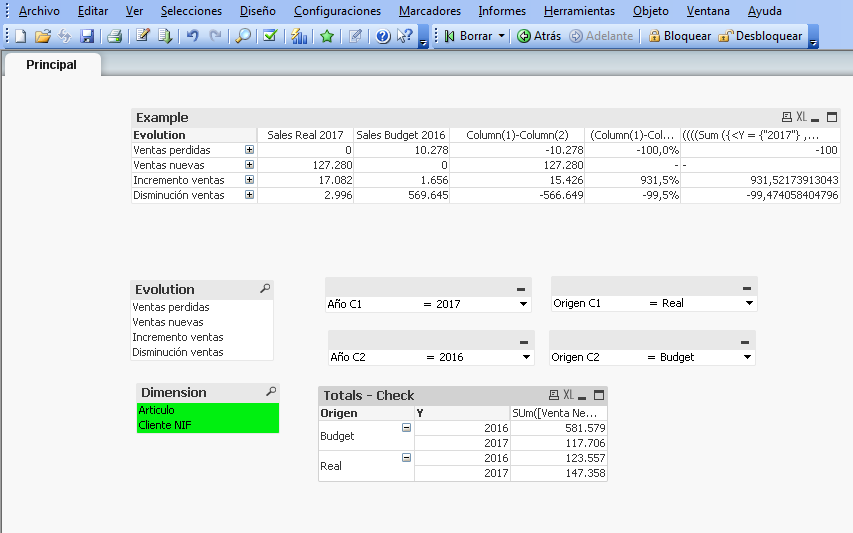# New to QlikView

Discussion board where members can get started with QlikView.

Announcements
Make your voice heard! Participate in the 2020 Wisdom of Crowds® Survey. BEGIN SURVEY
Highlighted
New Contributor III

## Variable dimensions

This must be easy I hope: I've create a variable vCritDimension which content is:

=If(GetSelectedCount(Dimension)>0,CONCAT(DISTINCT Dimension,  ', '))

Where Dimension is a result of:

Dimension

'Cliente NIF'

'Articulo'

];

I have a list Dimension and use the selected values to show or hide dimensions with the next condition, and it works:

=GetSelectedCount(Dimension)>0 and  SubStringCount( GetFieldSelections(Dimension), 'Cliente NIF')>=1

But when I use vCritDimension in an Aggr function it's not understood as dimensions... Do I miss something with \$ or whatever??

1 Solution

Accepted Solutions
HighlightedMVP

## Re: Variable dimensions

Also, created a new field in the script

Articulo,

[Cliente NIF],

[Grupo Cliente],

Articulo&[Cliente NIF] as [Articulo Cliente NIF],

Y,

M,

D,

[Venta Neta]

FROM

(ooxml, embedded labels);

Dimension

'Cliente NIF'

'Articulo'

];

12 Replies
HighlightedMVP

## Re: Variable dimensions

May be change your variable to this

=If(GetSelectedCount(Dimension)>0, Concat (DISTINCT '[' & Dimension & ']',  ', '))

and use your variable within a dollar sign expansion

\$(=vCritDimension) or \$(=\$(vCritDimension))

New Contributor III

## Re: Variable dimensions

Sunny, it works but have a problem:

If I use one (of the two available) dimension, and click on the calculated dimension, it's filtered correctly, but if I use two dimensions, the clicking/filtering behaviour is not correct. This is the formula used in the calculated dimension:

=Aggr(

If(Sum({<Y = {"\$(=[Año 1])"}, Origen = {"\$(=[Origen C1])"}>}[Venta Neta])=0 and sum({<Y = {"\$(=[Año 2])"}, Origen = {"\$(=[Origen C2])"}>}[Venta Neta])<>0,'Ventas perdidas',

If(Sum({<Y = {"\$(=[Año 2])"}, Origen = {"\$(=[Origen C2])"}>}[Venta Neta])=0 and sum({<Y = {"\$(=[Año 1])"}, Origen = {"\$(=[Origen C1])"}>}[Venta Neta])<>0,'Ventas nuevas',

If(Sum({<Y = {"\$(=[Año 1])"}, Origen = {"\$(=[Origen C1])"}>}[Venta Neta])>=Sum({<Y = {"\$(=[Año 2])"},Origen = {"\$(=[Origen C2])"}>}[Venta Neta]), 'Incremento ventas',

If(Sum({<Y = {"\$(=[Año 1])"}, Origen = {"\$(=[Origen C1])"}>}[Venta Neta])< Sum({<Y = {"\$(=[Año 2])"},Origen = {"\$(=[Origen C2])"}>}[Venta Neta]), 'Disminución ventas',

)))), \$(=vCritDimension))

It's only the clicking/filtering function, and I can live with it, but I don't understand it, showed information is correct, and I can expand and contract correctly... I just cannot follow the logic,when IO click some information is filtered, and other is not, but it seems that there's no logical explanation.

HighlightedMVP

## Re: Variable dimensions

 the clicking/filtering behaviour is not correct.

What exactly are you clicking/filtering? In the chart itself? Would you be able to share a sample to demonstrate the issue?

Highlighted
New Contributor III

## Re: Variable dimensions

Yes,. it is in the chart itself, on the output value in the calculated dimension column, I'll try to update a small example I have been using...

HighlightedMVP

## Re: Variable dimensions

Ya I can see why it would be a problem. Not sure if I have a solution for this problem.

Highlighted
New Contributor III

## Re: Variable dimensions

Eh? I can't find the attach function?!?!?!

Highlighted
New Contributor III

## Re: Variable dimensions

Here it is...Having [Articulo] y [Cliente NIF] selected, when you click in Example a calculated dimension, it doesn't work propperly...

HighlightedMVP

## Re: Variable dimensions

Checking...

HighlightedMVP

## Re: Variable dimensions

Not sure how many dimensions are possible, but you might have to do something like what I did to make it work....